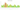# Ft

## Hungarian Forint

#### how has the value of the currency changed in the last year?## USD 1 = HUF 300.5

How much is10 US dollars worth inHungarian forints?
At the current exchange rate, 10 US dollars is worth 3,004.97 Hungarian forints
How much is50 US dollars worth inHungarian forints?
At the current exchange rate, 50 US dollars is worth 15,024.86 Hungarian forints
How much is100 US dollars worth inHungarian forints?
At the current exchange rate, 100 US dollars is worth 30,049.72 Hungarian forints
How much is500 US dollars worth inHungarian forints?
At the current exchange rate, 500 US dollars is worth 150,248.6 Hungarian forints
How much is2,000 US dollars worth inHungarian forints?
At the current exchange rate, 2,000 US dollars is worth 600,994.39 Hungarian forints
How much is10 US dollars worth inHungarian forints?
At the current exchange rate, 10 US dollars is worth 3,004.97 Hungarian forints
How much is50 US dollars worth inHungarian forints?
At the current exchange rate, 50 US dollars is worth 15,024.86 Hungarian forints
How much is100 US dollars worth inHungarian forints?
At the current exchange rate, 100 US dollars is worth 30,049.72 Hungarian forints
How much is500 US dollars worth inHungarian forints?
At the current exchange rate, 500 US dollars is worth 150,248.6 Hungarian forints
How much is2,000 US dollars worth inHungarian forints?
At the current exchange rate, 2,000 US dollars is worth 600,994.39 Hungarian forints